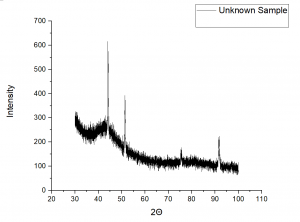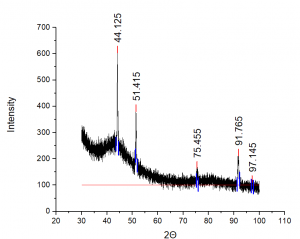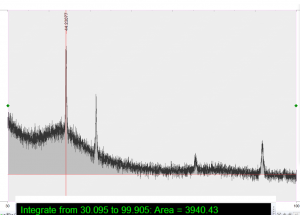X-ray diffraction experiments can be explained by Bragg’s law. The reflected x-rays from long-range crystallographic order planes can be predicted using the following equation.$n\lambda=2d\sin(\theta)$

Where n is an integer, λ is the wavelength of the incident x-rays, d is the inter-atomic spacing, and θ is the angle of incidence.

When the conditions of this equation are met, the x-rays that are diffracted off the material produce constructive interference, which causes high-intensity peaks on the spectrum. Semi-crystalline materials are essentially 2-phase systems with one phase being crystalline and the other being amorphous. Bragg’s law most accurately represents crystalline samples; when amorphous material is present in the sample, it disrupts the long-range order. When amorphous phases are present, they exhibit broad features based on the distribution of interatomic distances within the disordered structure. These broad features are called a halo hump or amorphous halo.

Integration Method analysis

The integration method uses a straight background line and compares the area under the entire curve with the area under the crystalline peaks using the following equation.

%Crystallinity = [(area under the crystalline peaks)/(area under all peaks)] *100

### Analysis

The following analysis can be done (to our knowledge) by any XRD-pattern analysis software. This example uses Origin 2020.

Collect your sample’s diffraction pattern over the desired 2theta range. If your sample is semi-crystalline or fully amorphous, you’ll see a broad feature, as seen in this figure between 35 and 55 2theta.Determine the area under the crystalline peaks, and record that value. The background should follow the shape of the noise (including the amorphous halo).Using a straight-line background, find the area under the entire curve.Using these values, the %crystallinity equation given above can be used to calculate approximate the crystalline and amorphous content of the sample. In this case, the area under the crystalline peaks was 470.13 and the total area was 3940. The sample had a crystallinity of 12% (meaning 88% amorphous material.